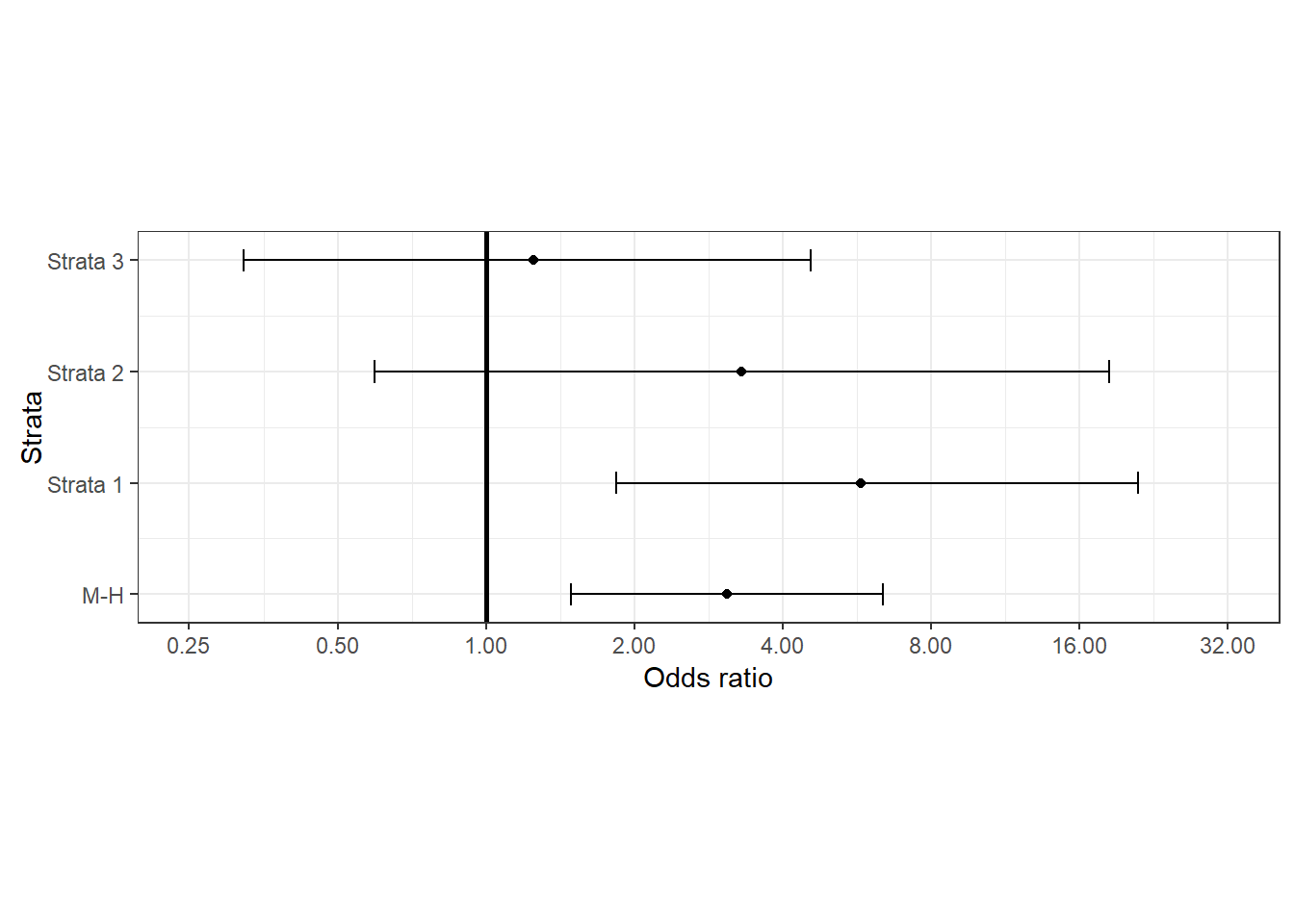# Measures of Association

#### 2023-01-15

A common task in epidemiology is to quantify the strength of association between exposures (‘risk factors’) and disease outcomes. In this context the term ‘exposure’ is taken to mean a variable whose association with the outcome is to be estimated.

Exposures can be harmful, beneficial or both harmful and beneficial (e.g., if an immunisable disease is circulating, exposure to immunising agents helps most recipients but may harm those who experience adverse reactions). The term ‘outcome’ is used to describe all the possible results that may arise from exposure to a causal factor or from preventive or therapeutic interventions . In human and animal health an ‘outcome-positive’ individual is an individual who has experienced a given disease of interest.

In this vignette we outline describe how epiR can be used to compute the various measures of association used in epidemiology notably the risk ratio, odds ratio, attributable risk in the exposed, attributable fraction in the exposed, attributable risk in the population and attributable fraction in the population. Examples are provided to demonstrate how the package can be used to deal with exposure-outcome data presented in various formats.

This vignette has been written assuming the reader routinely formats their 2 $$\times$$ 2 table data with the outcome status as columns and exposure status as rows. If this is not the case the argument outcome = "as.columns" (the default) can be changed to outcome = "as.rows".

Measures of association strength

The incidence risk ratio

Consider a study where subjects are disease free at the start of the study and all are monitored for disease occurrence for a specified time period. At the start of the study period study subjects are classified according to exposure to a hypothesised risk factor. If both exposure and outcome are binary variables (yes or no) we can present the counts of subjects in each of the four exposure-disease categories in a 2 $$\times$$ 2 table.

Table 1: A 2 x 2 contingency table.

Dis +

Dis -

Total

Exp +

a

b

a + b

Exp -

c

c

c + d

Total

a + c

b + c

a + b + c + d

When our data are in this format we can calculate the incidence risk of the outcome in those that were exposed $$R_E+$$, the incidence risk in those that were not exposed $$R_{E-}$$ and finally the incidence risk in the total study population $$R_{T}$$:

Table 2: A 2 x 2 table with incidence risks calculated for the exposed, the unexposed and the total study population.

Dis +

Dis -

Total

Risk

a

b

a + b

RE+ = a / (a + b)

Exp +

c

c

c + d

RE- = c / (c + d)

Exp -

a + c

b + c

a + b + c + d

RT = (a + c) / (a + b + c + d)

The incidence risk estimates shown in Table 2 can be presented graphically in the format shown in Figure 1. In Figure 1 we present the calculated incidence risk in the exposed, unexposed and (exposed and unexposed) groups as a bar chart. The incidence risk ratio equals the incidence risk of the outcome in the exposed divided by the incidence risk of the outcome in the unexposed: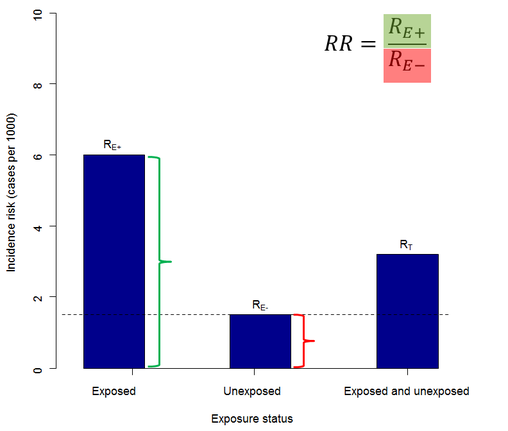The incidence risk ratio.

The incidence risk ratio provides an estimate of how many times more likely exposed individuals are to experience the outcome of interest, compared with non-exposed individuals.

If the incidence risk ratio equals 1, then the risk of the outcome in both the exposed and non-exposed groups are equal. If the incidence risk ratio is greater than 1, then exposure increases the outcome risk with greater departures from 1 indicative of a stronger effect. If the incidence risk ratio is less than 1, exposure reduces the outcome risk and exposure is said to be protective.

The odds ratio — cohort studies

In a cohort study definition of exposure status (exposure-positive, exposure-negative) comes first. Subjects are then followed over time to determine their outcome status (outcome-positive, outcome-negative). The odds of the outcome in the exposed and unexposed populations are calculated as follows:

Table 3: A 2 x 2 table with the odds of disease calculated for the exposed, the unexposed and the total study population.

Dis +

Dis -

Total

Odds

a

b

a + b

OE+ = a / b

Exp +

c

d

c + d

OE- = c / d

Exp -

a + c

b + d

a + b + c + d

OT = (a + c) / (b + d)

The odds ratio for a cohort study is then the odds of the outcome in the exposed divided by the odds of the outcome in the unexposed.

The odds ratio — case-control studies

In a case-control study outcome status (disease-positive, disease-negative) is defined first. The history provided by each study subject then provides information about exposure status. For case-control studies, instead of talking about the odds of disease in the exposed and unexposed groups (as we did when we were working with data from a cohort study) we talk about the odds of exposure in the case and control groups.

Table 4: A 2 x 2 table with the odds of exposure calculated for cases, controls and the total study population.

Case

Control

Total

Exp +

a

b

a + b

Exp -

c

d

c + d

Odds

OD+ = a / c

OD- = b / d

OT = (a + b) / (c + d)

The odds ratio is defined as the odds of exposure in the cases ($$O_{D+}$$) divided by the odds of exposure in the controls ($$O_{D-}$$).

Note that the numeric estimate of the odds ratio is exactly the same as that calculated for a cohort study. The expression of the result is the only thing that differs. Key points:

• In a cohort study we talk about the odds of disease being $$x$$ times greater (or less) in the exposed, compared with the unexposed.

• In a case-control study we talk about the odds of exposure being $$x$$ times greater (or less) in cases, compared with controls.

Measures of effect in the exposed

The attributable risk in the exposed

The attributable risk is defined as the increase or decrease in the risk of the outcome in the exposed that is attributable to exposure (Figure 2). Attributable risk (unlike the incidence risk ratio) provides a measure of the absolute frequency of the outcome associated with exposure.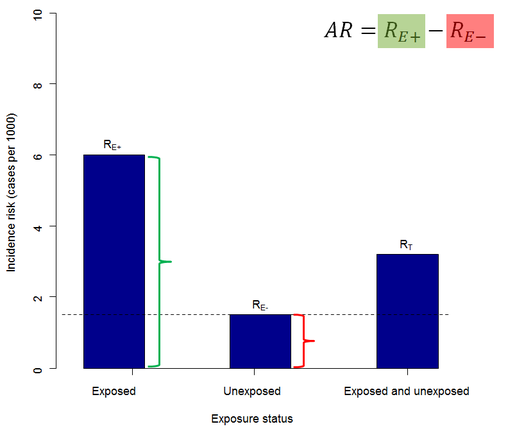The attributable risk in the exposed.

A useful way of expressing attributable risk in a clinical setting is in terms of the number needed to treat, NNT. NNT equals the inverse of the attributable risk. Depending on the outcome of interest we often elect to use different labels for NNT.

When dealing with an outcome that is ‘desirable’ (e.g., treatment success) we call NNT the number needed to treat for benefit, NNTB. NNTB equals the number of subjects who would have to be exposed to result in a single (desirable) outcome. When dealing with an outcome that is ‘undesirable’ (e.g., death) we call NNT the number needed to treat for harm, NNTH. NNTH equals the number of subjects who would have to be exposed to result in a single (undesirable) outcome.

The attributable fraction in the exposed

The attributable fraction in the exposed is the proportion of outcome-positive subjects in the exposed group that is due to exposure (Figure 3).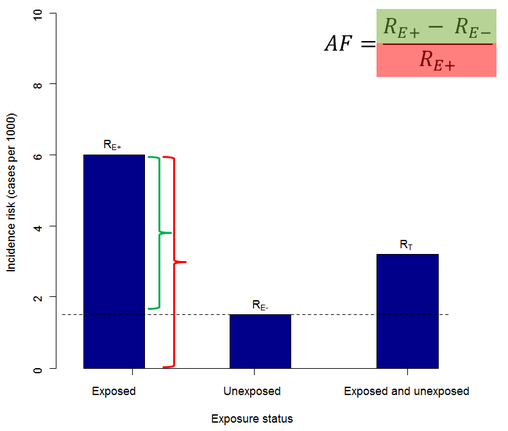The attributable fraction in the exposed.

Measures of effect in the population

The attributable risk in the population

The population attributable risk is the increase or decrease in incidence risk of the outcome in the study population that is attributable to exposure (Figure 4).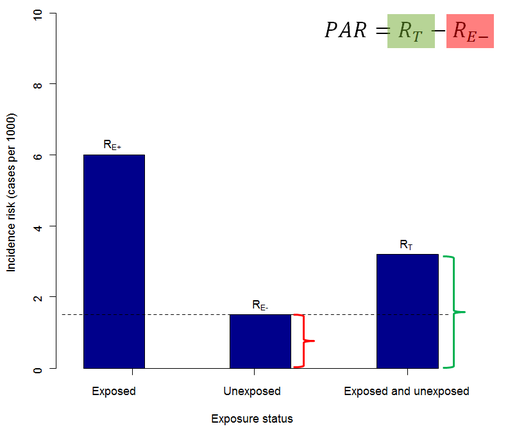The attributable risk in the population

The attributable fraction in the population

The population attributable fraction (also known as the aetiologic fraction) is the proportion of outcome-positive subjects in the study population that is due to the exposure (Figure 5).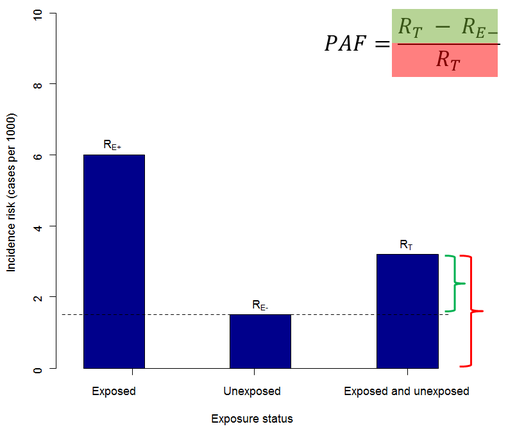The attributable fraction in the population

On the condition that the exposure of interest is a cause of the disease outcome, the population attributable fraction represents the proportional reduction in average disease risk over a specified period of time that would be achieved by eliminating the exposure of interest while the distribution of other risk factors in the population remained unchanged. For this reason, PAFs are particularly useful to guide policy makers when planning public health interventions. If you’re going to use PAFs as a means for informing policy, make sure that: (1) the exposure of interest is causally related to the outcome, and (2) the exposure of interest is something amenable to intervention.

Theory to practice: Calculating measures of association using R

Direct entry of 2 by 2 table contingency table cell frequencies

Firstly, a 2 $$\times$$ 2 table can be created by listing the contingency table cell frequencies in vector format. Take the following example.

A cross sectional study investigating the relationship between dry cat food (DCF) and feline lower urinary tract disease (FLUTD) was conducted . Counts of individuals in each group were as follows. DCF-exposed cats (cases, non-cases) 13, 2163. Non DCF-exposed cats (cases, non-cases) 5, 3349. We can enter these data directly into R as a vector of length four. Check that your counts have been entered in the correct order by viewing the data as a matrix.

dat.v01 <- c(13,2163,5,3349); dat.v01
#>    13 2163    5 3349

# View the data in the usual 2 by 2 table format:
matrix(dat.v01, nrow = 2, byrow = TRUE)
#>      [,1] [,2]
#> [1,]   13 2163
#> [2,]    5 3349

Calculate the prevalence ratio, odds ratio, attributable prevalence in the exposed, attributable fraction in the exposed, attributable prevalence in the population and the attributable fraction in the population using function epi.2by2. Note that we use the term prevalence ratio (instead of incidence risk ratio) here because we’re dealing with data from a cross-sectional study — the frequency of disease is expressed as a prevalence, not an incidence.

library(epiR)

epi.2by2(dat = dat.v01, method = "cross.sectional", conf.level = 0.95, units = 100,
interpret = FALSE, outcome = "as.columns")
#>              Outcome +    Outcome -      Total               Prevalence *
#> Exposed +           13         2163       2176        0.60 (0.32 to 1.02)
#> Exposed -            5         3349       3354        0.15 (0.05 to 0.35)
#> Total               18         5512       5530        0.33 (0.19 to 0.51)
#>
#> Point estimates and 95% CIs:
#> -------------------------------------------------------------------
#> Prevalence ratio                               4.01 (1.43, 11.23)
#> Odds ratio                                     4.03 (1.43, 11.31)
#> Attrib prevalence in the exposed *             0.45 (0.10, 0.80)
#> Attrib fraction in the exposed (%)            75.05 (30.11, 91.09)
#> Attrib prevalence in the population *          0.18 (-0.02, 0.38)
#> Attrib fraction in the population (%)         54.20 (3.61, 78.24)
#> -------------------------------------------------------------------
#> Uncorrected chi2 test that OR = 1: chi2(1) = 8.177 Pr>chi2 = 0.004
#> Fisher exact test that OR = 1: Pr>chi2 = 0.006
#>  Wald confidence limits
#>  CI: confidence interval
#>  * Outcomes per 100 population units

The prevalence of FLUTD in DCF exposed cats was 4.01 (95% CI 1.43 to 11.23) times greater than the prevalence of FLUTD in non-DCF exposed cats. In DCF exposed cats, 75% of FLUTD was attributable to DCF (95% CI 30% to 91%). Fifty-four percent of FLUTD cases in this cat population were attributable to DCF (95% CI 4% to 78%).

Need a hand getting the correct wording to explain each of the listed measures of association and measures of effect? Set interpret = TRUE in epi.2by2:

epi.2by2(dat = dat.v01, method = "cross.sectional", conf.level = 0.95, units = 100,
interpret = TRUE, outcome = "as.columns")
#>              Outcome +    Outcome -      Total               Prevalence *
#> Exposed +           13         2163       2176        0.60 (0.32 to 1.02)
#> Exposed -            5         3349       3354        0.15 (0.05 to 0.35)
#> Total               18         5512       5530        0.33 (0.19 to 0.51)
#>
#> Point estimates and 95% CIs:
#> -------------------------------------------------------------------
#> Prevalence ratio                               4.01 (1.43, 11.23)
#> Odds ratio                                     4.03 (1.43, 11.31)
#> Attrib prevalence in the exposed *             0.45 (0.10, 0.80)
#> Attrib fraction in the exposed (%)            75.05 (30.11, 91.09)
#> Attrib prevalence in the population *          0.18 (-0.02, 0.38)
#> Attrib fraction in the population (%)         54.20 (3.61, 78.24)
#> -------------------------------------------------------------------
#> Uncorrected chi2 test that OR = 1: chi2(1) = 8.177 Pr>chi2 = 0.004
#> Fisher exact test that OR = 1: Pr>chi2 = 0.006
#>  Wald confidence limits
#>  CI: confidence interval
#>  * Outcomes per 100 population units
#>
#>  Measures of association strength:
#>  The outcome prevalence among the exposed was 4.01 (95% CI 1.43 to 11.23) times greater than the outcome prevalence among the unexposed.
#>
#>  The outcome odds among the exposed was 4.03 (95% CI 1.43 to 11.31) times greater than the outcome odds among the unexposed.
#>
#>  Measures of effect in the exposed:
#>  Exposure changed the outcome prevalence in the exposed by 0.45 (95% CI 0.1 to 0.8) per 100 population units. 75.2% of outcomes in the exposed were attributable to exposure (95% CI 25.7% to 93.1%).
#>
#>  Number needed to treat for benefit (NNTB) and harm (NNTH):
#>  The number needed to treat for one subject to benefit (NNTB) is 223 (95% CI 125 to 1008).
#>
#>  Measures of effect in the population:
#>  Exposure changed the outcome prevalence in the population by 0.18 (95% CI -0.02 to 0.38) per 100 population units. 54.3% of outcomes in the population were attributable to exposure (95% CI 32.5% to 75%).

Data frame with one row per observation

Here we provide examples where you have exposure status and outcome status listed for each member of your study population. There are two options for contingency table preparation in this situation: (1) using base R’s table function; or (2) using the tidyverse package.

For this example we use the low infant birth weight data presented by Hosmer and Lemeshow (2000) and available in the MASS package in R. The birthwt data frame has 189 rows and 10 columns. The data were collected at Baystate Medical Center, Springfield, Massachusetts USA during 1986.

Two by two table preparation using the table function in base R

library(MASS)

# Load and view the data:
#>    low age lwt race smoke ptl ht ui ftv  bwt
#> 85   0  19 182    2     0   0  0  1   0 2523
#> 86   0  33 155    3     0   0  0  0   3 2551
#> 87   0  20 105    1     1   0  0  0   1 2557
#> 88   0  21 108    1     1   0  0  1   2 2594
#> 89   0  18 107    1     1   0  0  1   0 2600
#> 91   0  21 124    3     0   0  0  0   0 2622

Each row of this data set represents data for one mother. We’re interested in the association between smoke (the mother’s smoking status during pregnancy) and low (delivery of a baby less than 2.5 kg bodyweight).

Its important that the table you present to epi.2by2 is in the correct format: Outcome positives in the first column, outcome negatives in the second column, exposure positives in the first row and exposure negatives in the second row. If we run the table function on the bwt data the output table is in the wrong format:

dat.tab02 <- table(dat.df02$smoke, dat.df02$low, dnn = c("Smoke", "Low BW")); dat.tab02
#>      Low BW
#> Smoke  0  1
#>     0 86 29
#>     1 44 30

There are two ways to fix this problem. The quick fix is to simply ask R to switch the order of the rows and columns in the output table:

dat.tab02 <- table(dat.df02$smoke, dat.df02$low, dnn = c("Smoke", "Low BW")); dat.tab02
#>      Low BW
#> Smoke  0  1
#>     0 86 29
#>     1 44 30
dat.tab02 <- dat.tab02[2:1,2:1]; dat.tab02
#>      Low BW
#> Smoke  1  0
#>     1 30 44
#>     0 29 86

The second approach is to set the exposure variable and the outcome variable as a factor and to define the levels of each factor using levels = c(1,0):

# Variables low, smoke and race as factors. Put an 'f' in front of the variable names to remind you that they're factors:

dat.df02$flow <- factor(dat.df02$low, levels = c(1,0))
dat.df02$fsmoke <- factor(dat.df02$smoke, levels = c(1,0))
dat.df02$frace <- factor(dat.df02$race, levels = c(1,2,3))

dat.tab02 <- table(dat.df02$fsmoke, dat.df02$flow, dnn = c("Smoke", "Low BW")); dat.tab02
#>      Low BW
#> Smoke  1  0
#>     1 30 44
#>     0 29 86

Now compute the odds ratio for smoking and delivery of a low birth weight baby:

dat.epi02 <- epi.2by2(dat = dat.tab02, method = "cohort.count", conf.level = 0.95,
units = 100, interpret = FALSE, outcome = "as.columns")
dat.epi02
#>              Outcome +    Outcome -      Total                 Inc risk *
#> Exposed +           30           44         74     40.54 (29.27 to 52.59)
#> Exposed -           29           86        115     25.22 (40.54 to 40.54)
#> Total               59          130        189     31.22 (24.69 to 38.35)
#>
#> Point estimates and 95% CIs:
#> -------------------------------------------------------------------
#> Inc risk ratio                                 1.61 (1.06, 2.44)
#> Odds ratio                                     2.02 (1.08, 3.78)
#> Attrib risk in the exposed *                   15.32 (1.61, 29.04)
#> Attrib fraction in the exposed (%)            37.80 (5.47, 59.07)
#> Attrib risk in the population *                6.00 (-4.33, 16.33)
#> Attrib fraction in the population (%)         19.22 (-0.21, 34.88)
#> -------------------------------------------------------------------
#> Uncorrected chi2 test that OR = 1: chi2(1) = 4.924 Pr>chi2 = 0.026
#> Fisher exact test that OR = 1: Pr>chi2 = 0.036
#>  Wald confidence limits
#>  CI: confidence interval
#>  * Outcomes per 100 population units

The odds of having a low birth weight child for smokers is 2.02 (95% CI 1.08 to 3.78) times greater than the odds of having a low birth weight child for non-smokers. All of the calculated measures of association computed by epi.2by2 can be listed using:

names(dat.epi02$massoc.detail) #>  "RR.strata.wald" "RR.strata.taylor" "RR.strata.score" #>  "OR.strata.wald" "OR.strata.cfield" "OR.strata.score" #>  "OR.strata.mle" "ARisk.strata.wald" "ARisk.strata.score" #>  "NNT.strata.wald" "NNT.strata.score" "AFRisk.strata.wald" #>  "PARisk.strata.wald" "PARisk.strata.piri" "PAFRisk.strata.wald" #>  "chi2.strata.uncor" "chi2.strata.yates" "chi2.strata.fisher" #>  "chi2.correction" Compare the Wald confidence intervals for the odds ratio with the score confidence intervals: dat.epi02$massoc.detail$OR.strata.wald #> est lower upper #> 1 2.021944 1.08066 3.783112 # Wald confidence intervals: 2.02 (95% CI 1.08 to 3.78) dat.epi02$massoc.detail$OR.strata.score #> est lower upper #> 1 2.021944 1.084385 3.773885 # Score confidence intervals: 2.02 (95% CI 1.08 to 3.77) Two by two table preparation using tidyverse The tidyverse package can also be used to prepare data in the required format: library(tidyverse) dat.df03 <- birthwt; head(dat.df03) #> low age lwt race smoke ptl ht ui ftv bwt #> 85 0 19 182 2 0 0 0 1 0 2523 #> 86 0 33 155 3 0 0 0 0 3 2551 #> 87 0 20 105 1 1 0 0 0 1 2557 #> 88 0 21 108 1 1 0 0 1 2 2594 #> 89 0 18 107 1 1 0 0 1 0 2600 #> 91 0 21 124 3 0 0 0 0 0 2622 # Here we set the factor levels and tabulate the data in a single call using pipe operators: dat.tab03 <- dat.df03 %>% mutate(flow = factor(low, levels = c(1,0), labels = c("yes","no"))) %>% mutate(fsmoke = factor(smoke, levels = c(1,0), labels = c("yes","no"))) %>% group_by(fsmoke, flow) %>% summarise(n = n()) # View the data: dat.tab03 #> # A tibble: 4 × 3 #> # Groups: fsmoke  #> fsmoke flow n #> <fct> <fct> <int> #> 1 yes yes 30 #> 2 yes no 44 #> 3 no yes 29 #> 4 no no 86 # View the data in conventional 2 by 2 table format: pivot_wider(dat.tab03, id_cols = c(fsmoke), names_from = flow, values_from = n) #> # A tibble: 2 × 3 #> # Groups: fsmoke  #> fsmoke yes no #> <fct> <int> <int> #> 1 yes 30 44 #> 2 no 29 86 As before, compute the odds ratio for smoking and delivery of a low birth weight baby: dat.epi03 <- epi.2by2(dat = dat.tab03, method = "cohort.count", conf.level = 0.95, units = 100, interpret = FALSE, outcome = "as.columns") dat.epi03 #> Outcome + Outcome - Total Inc risk * #> Exposed + 30 44 74 40.54 (29.27 to 52.59) #> Exposed - 29 86 115 25.22 (40.54 to 40.54) #> Total 59 130 189 31.22 (24.69 to 38.35) #> #> Point estimates and 95% CIs: #> ------------------------------------------------------------------- #> Inc risk ratio 1.61 (1.06, 2.44) #> Odds ratio 2.02 (1.08, 3.78) #> Attrib risk in the exposed * 15.32 (1.61, 29.04) #> Attrib fraction in the exposed (%) 37.80 (5.47, 59.07) #> Attrib risk in the population * 6.00 (-4.33, 16.33) #> Attrib fraction in the population (%) 19.22 (-0.21, 34.88) #> ------------------------------------------------------------------- #> Uncorrected chi2 test that OR = 1: chi2(1) = 4.924 Pr>chi2 = 0.026 #> Fisher exact test that OR = 1: Pr>chi2 = 0.036 #> Wald confidence limits #> CI: confidence interval #> * Outcomes per 100 population units The odds of having a low birth weight child for smokers is 2.02 (95% CI 1.08 to 3.78) times greater than the odds of having a low birth weight child for non-smokers. Measures of association for a series of candidate risk factors The code that follows provides and approach for calculating measures of association for a series of candidate risk factors, writing the results to a data frame. Here we set up a loop to calculate the odds ratio for age, smoke and race: dat.df04 <- birthwt; head(dat.df04) #> low age lwt race smoke ptl ht ui ftv bwt #> 85 0 19 182 2 0 0 0 1 0 2523 #> 86 0 33 155 3 0 0 0 0 3 2551 #> 87 0 20 105 1 1 0 0 0 1 2557 #> 88 0 21 108 1 1 0 0 1 2 2594 #> 89 0 18 107 1 1 0 0 1 0 2600 #> 91 0 21 124 3 0 0 0 0 0 2622 dat.df04$flow <- factor(dat.df04$low, levels = c(1,0)) dat.df04$fage <- ifelse(dat.df04$age > 23, 0,1) dat.df04$fage <- factor(dat.df04$fage, levels = c(1,0)) dat.df04$fsmoke <- factor(dat.df04$smoke, levels = c(1,0)) # Race is coded 1 = white, 2 = black and 3 = other. Set white as the reference level: dat.df04$frace <- ifelse(dat.df04$race == 1, 0, 1) dat.df04$frace <- factor(dat.df04$frace, levels = c(1,0)) # Empty vectors to collect results: rfactor <- ref <- or.p <- or.l <- or.u <- c() # The candidate risk factors are in columns 12 to 14 of data frame dat.df04: for(i in 12:14){ tdat.tab04 <- table(dat.df04[,i], dat.df04$flow)
tdat.epi04 <- epi.2by2(dat = tdat.tab04, method = "cohort.count",
conf.level = 0.95, units = 100, interpret = FALSE, outcome = "as.columns")

trfactor <- as.character(names(dat.df04)[i])
rfactor <- c(rfactor, trfactor)

tref <- as.character(paste("Reference: ", trfactor, " - ", levels(dat.df04[,i]), sep = ""))
ref <- c(ref, tref)

tor.p <- as.numeric(tdat.epi04$massoc.detail$OR.strata.wald)
or.p <- c(or.p, tor.p)

tor.l <- as.numeric(tdat.epi04$massoc.detail$OR.strata.wald)
or.l <- c(or.l, tor.l)

tor.u <- as.numeric(tdat.epi04$massoc.detail$OR.strata.wald)
or.u <- c(or.u, tor.u)
}

gdat.df04 <- data.frame(yat = 1:3, ylab = rfactor, ref, or.p, or.l, or.u)
gdat.df04
#>   yat   ylab                   ref     or.p      or.l     or.u
#> 1   1   fage   Reference: fage - 0 1.174769 0.6294241 2.192609
#> 2   2 fsmoke Reference: fsmoke - 0 2.021944 1.0806596 3.783112
#> 3   3  frace  Reference: frace - 0 2.004577 1.0703040 3.754380

Plot the point estimate of the odds ratio for each candidate risk factor and its 95% confidence interval. Annotate the plot with the reference category for each risk factor:

library(ggplot2); library(scales)

x.at <- c(0.25,0.5,1,2,4,8,16,32)

ggplot(data = gdat.df04, aes(x = or.p, y = yat)) +
theme_bw() +
geom_point() +
geom_errorbarh(aes(xmax = or.l, xmin = or.u, height = 0.2)) +
scale_x_continuous(trans = log2_trans(), breaks = x.at, limits = c(0.25,8),
name = "Odds ratio") +
scale_y_continuous(breaks = gdat.df04$yat, labels = gdat.df04$ylab,
name = "Risk factor") +
geom_vline(xintercept = 1, lwd = 1) +
annotate("text", x = 0.25, y = gdat.df04$yat, label = gdat.df04$ref, hjust = 0, size = 3) +
coord_fixed(ratio = 0.75 / 1) +
theme(axis.title.y = element_text(vjust = 0))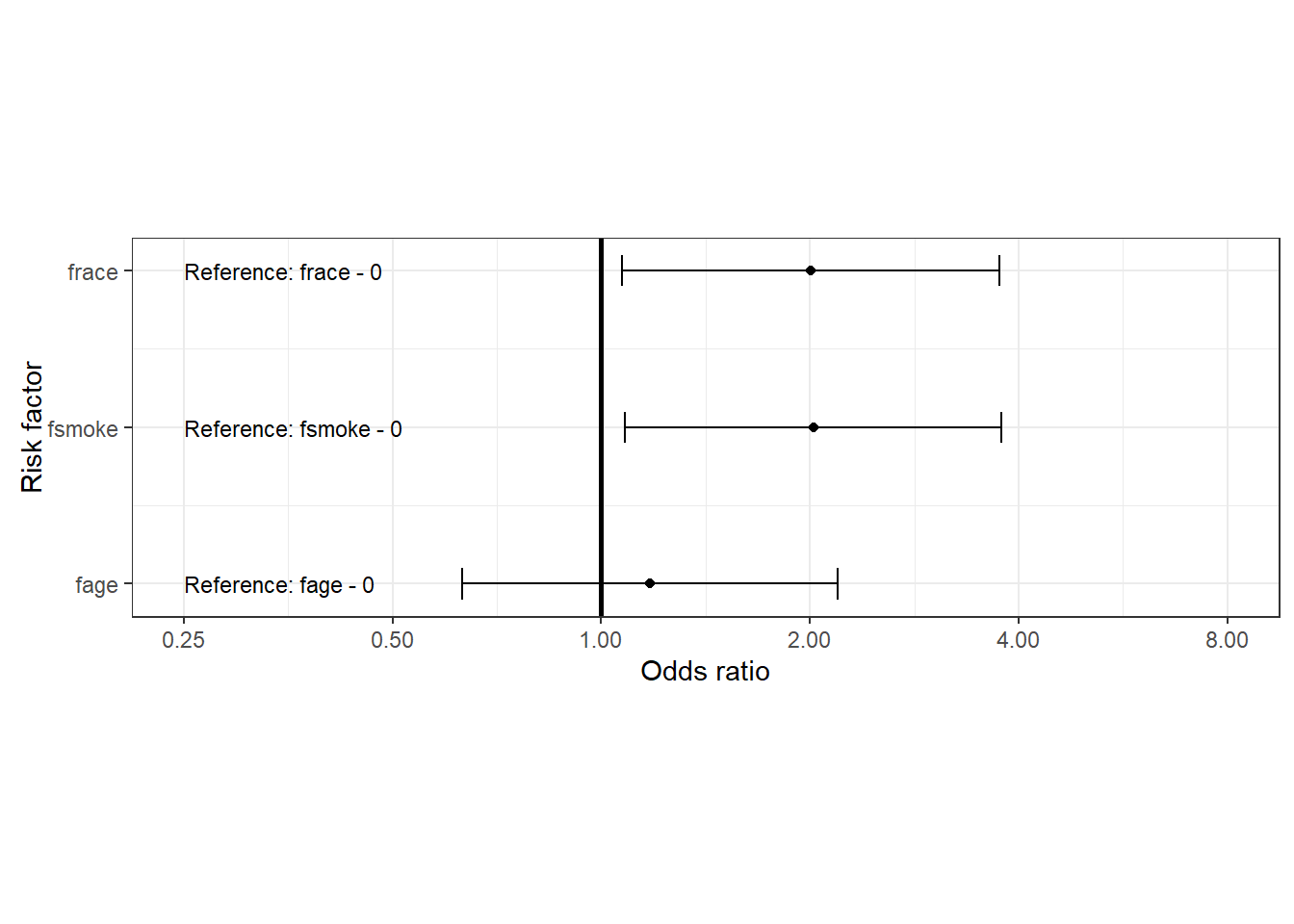Confounding

We’re concerned that the mother’s race may confound the association between low birth weight and delivery of a low birth weight baby so we’ll stratify the data by race and compute the Mantel-Haenszel adjusted odds ratio. As before, our tables can be prepared using either base R or tidyverse.

Stratified two by two table preparation using the table function in base R

dat.df05 <- birthwt; head(dat.df05)
#>    low age lwt race smoke ptl ht ui ftv  bwt
#> 85   0  19 182    2     0   0  0  1   0 2523
#> 86   0  33 155    3     0   0  0  0   3 2551
#> 87   0  20 105    1     1   0  0  0   1 2557
#> 88   0  21 108    1     1   0  0  1   2 2594
#> 89   0  18 107    1     1   0  0  1   0 2600
#> 91   0  21 124    3     0   0  0  0   0 2622

dat.df05$flow <- factor(dat.df05$low, levels = c(1,0))

dat.df05$fsmoke <- factor(dat.df05$smoke, levels = c(1,0))
dat.df05$frace <- factor(dat.df05$race, levels = c(1,2,3))

dat.tab05 <- table(dat.df05$fsmoke, dat.df05$flow, dat.df05$frace, dnn = c("Smoke", "Low BW", "Race")); dat.tab05 #> , , Race = 1 #> #> Low BW #> Smoke 1 0 #> 1 19 33 #> 0 4 40 #> #> , , Race = 2 #> #> Low BW #> Smoke 1 0 #> 1 6 4 #> 0 5 11 #> #> , , Race = 3 #> #> Low BW #> Smoke 1 0 #> 1 5 7 #> 0 20 35 Compute the Mantel-Haenszel adjusted odds ratio for smoking and delivery of a low birth weight baby, adjusting for the effect of race. Function epi.2by2 automatically calculates the Mantel-Haenszel odds ratio and risk ratio when presented with stratified contingency tables. dat.epi05 <- epi.2by2(dat = dat.tab05, method = "cohort.count", conf.level = 0.95, units = 100, interpret = FALSE, outcome = "as.columns") dat.epi05 #> Outcome + Outcome - Total Inc risk * #> Exposed + 30 44 74 40.54 (29.27 to 52.59) #> Exposed - 29 86 115 25.22 (40.54 to 40.54) #> Total 59 130 189 31.22 (24.69 to 38.35) #> #> #> Point estimates and 95% CIs: #> ------------------------------------------------------------------- #> Inc risk ratio (crude) 1.61 (1.06, 2.44) #> Inc risk ratio (M-H) 2.15 (1.29, 3.58) #> Inc risk ratio (crude:M-H) 0.75 #> Odds ratio (crude) 2.02 (1.08, 3.78) #> Odds ratio (M-H) 3.09 (1.49, 6.39) #> Odds ratio (crude:M-H) 0.66 #> Attrib risk in the exposed (crude) * 15.32 (1.61, 29.04) #> Attrib risk in the exposed (M-H) * 22.17 (1.41, 42.94) #> Attrib risk (crude:M-H) 0.69 #> ------------------------------------------------------------------- #> M-H test of homogeneity of IRRs: chi2(2) = 3.862 Pr>chi2 = 0.145 #> M-H test of homogeneity of ORs: chi2(2) = 2.800 Pr>chi2 = 0.247 #> Test that M-H adjusted OR = 1: chi2(1) = 9.413 Pr>chi2 = 0.001 #> Wald confidence limits #> M-H: Mantel-Haenszel; CI: confidence interval #> * Outcomes per 100 population units The Mantel-Haenszel test of homogeneity of the strata odds ratios is not significant (chi square test statistic 2.800; df 2; p-value = 0.25). We accept the null hypothesis and conclude that the odds ratios for each strata of race are the same. Because the stratum specific odds ratios are not significantly different the Mantel-Haenszel adjusted odds ratio provides an appropriate summary of the association between smoking and low birth weight. After accounting for the confounding effect of race, the odds of having a low birth weight baby for smokers was 3.09 (95% CI 1.49 to 6.39) times that of non-smokers. Stratified two by two table preparation using tidyverse dat.df06 <- birthwt dat.tab06 <- dat.df06 %>% mutate(flow = factor(low, levels = c(1,0), labels = c("yes","no"))) %>% mutate(fsmoke = factor(smoke, levels = c(1,0), labels = c("yes","no"))) %>% mutate(frace = factor(race)) %>% group_by(frace, fsmoke, flow) %>% summarise(n = n()) dat.tab06 #> # A tibble: 12 × 4 #> # Groups: frace, fsmoke  #> frace fsmoke flow n #> <fct> <fct> <fct> <int> #> 1 1 yes yes 19 #> 2 1 yes no 33 #> 3 1 no yes 4 #> 4 1 no no 40 #> # … with 8 more rows # View the data in conventional 2 by 2 table format: pivot_wider(dat.tab06, id_cols = c(frace, fsmoke), names_from = flow, values_from = n) #> # A tibble: 6 × 4 #> # Groups: frace, fsmoke  #> frace fsmoke yes no #> <fct> <fct> <int> <int> #> 1 1 yes 19 33 #> 2 1 no 4 40 #> 3 2 yes 6 4 #> 4 2 no 5 11 #> # … with 2 more rows Compute the Mantel-Haenszel adjusted odds ratio for smoking and delivery of a low birth weight baby, adjusting for the effect of race: dat.epi06 <- epi.2by2(dat = dat.tab06, method = "cohort.count", conf.level = 0.95, units = 100, interpret = FALSE, outcome = "as.columns") dat.epi06 #> Outcome + Outcome - Total Inc risk * #> Exposed + 30 44 74 40.54 (29.27 to 52.59) #> Exposed - 29 86 115 25.22 (40.54 to 40.54) #> Total 59 130 189 31.22 (24.69 to 38.35) #> #> #> Point estimates and 95% CIs: #> ------------------------------------------------------------------- #> Inc risk ratio (crude) 1.61 (1.06, 2.44) #> Inc risk ratio (M-H) 2.15 (1.29, 3.58) #> Inc risk ratio (crude:M-H) 0.75 #> Odds ratio (crude) 2.02 (1.08, 3.78) #> Odds ratio (M-H) 3.09 (1.49, 6.39) #> Odds ratio (crude:M-H) 0.66 #> Attrib risk in the exposed (crude) * 15.32 (1.61, 29.04) #> Attrib risk in the exposed (M-H) * 22.17 (1.41, 42.94) #> Attrib risk (crude:M-H) 0.69 #> ------------------------------------------------------------------- #> M-H test of homogeneity of IRRs: chi2(2) = 3.862 Pr>chi2 = 0.145 #> M-H test of homogeneity of ORs: chi2(2) = 2.800 Pr>chi2 = 0.247 #> Test that M-H adjusted OR = 1: chi2(1) = 9.413 Pr>chi2 = 0.001 #> Wald confidence limits #> M-H: Mantel-Haenszel; CI: confidence interval #> * Outcomes per 100 population units Plot the individual strata odds ratios and the Mantel-Haenszel summary odds ratio as an error bar plot to better understand how the Mantel-Haenszel adjusted odds ratio relates to the individual strata odds ratios: nstrata <- 1:length(unique(dat.tab06$frace))
strata.lab <- paste("Strata ", nstrata, sep = "")
y.at <- c(nstrata, max(nstrata) + 1)
y.lab <- c("M-H", strata.lab)
x.at <- c(0.25,0.5,1,2,4,8,16,32)

or.p <- c(dat.epi06$massoc.detail$OR.mh$est, dat.epi06$massoc.detail$OR.strata.cfield$est)
or.l <- c(dat.epi06$massoc.detail$OR.mh$lower, dat.epi05$massoc.detail$OR.strata.cfield$lower)
or.u <- c(dat.epi06$massoc.detail$OR.mh$upper, dat.epi05$massoc.detail$OR.strata.cfield$upper)
gdat.df06 <- data.frame(y.at, y.lab, or.p, or.l, or.u)

ggplot(data = gdat.df06, aes(x = or.p, y = y.at)) +
theme_bw() +
geom_point() +
geom_errorbarh(aes(xmax = or.l, xmin = or.u, height = 0.2)) +
labs(x = "Odds ratio", y = "Strata") +
scale_x_continuous(trans = log2_trans(), breaks = x.at,
limits = c(0.25,32)) +
scale_y_continuous(breaks = y.at, labels = y.lab) +
geom_vline(xintercept = 1, lwd = 1) +
coord_fixed(ratio = 0.75 / 1) +
theme(axis.title.y = element_text(vjust = 0))